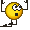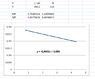# Calculate the boiling temperature of methanol from thermodynamic data

• Chemistry
il postino
Homework Statement:
Calculate the boiling temperature of methanol at 60 atm knowing that Tc = 513K, Pc = 78atm and the acentricity is W.
Relevant Equations:
Pitzer correlation?
Clausius equation?
Calculate the boiling temperature of methanol at 60 atm knowing that Tc = 513K, Pc = 78 atm and the acentricity is 0.555.

I would like you to help me start the exercise.
I thought about using the Pitzer Correlation to be able to calculate the fugacity coefficient, but I don't have the Temperature.
I thought about using, then, the Clausius equation but I don't have the enthalpy either.
I don't know how to start.
Thank you very much!

Mentor
The acentric factor is defined by the equation $$\omega=-1-\log{(P_r^{sat})_{T_r=0.7}}$$What value does this give you for ##(P_r^{sat})_{T_r=0.7}##?

Last edited:
•il postino
il postino
The acentric factor is defined by the equation $$\omega=-1-\log{(P_r^{sat})_{T_r=0.7}}$$What value does this give you for ##(P_r^{sat})_{T_r=0.7}##?
Hi! Thank you very much for answering!
I have not been taught this formula.
How can I apply it to solve the exercise?

Last edited by a moderator:
Mentor
Hi! Thank you very much for answering!
I have not been taught this formula.
How can I apply it to solve the exercise?

il postino
[QUOTE = "Chestermiller, publicación: 6368754, miembro: 345636"]
The acentric factor is defined by the equation $$\omega=-1-\log{(P_r^{sat})_{T_r=0.7}}$$What value does this give you for ##(P_r^{sat})_{T_r=0.7}##?
[/QUOTE]

But how do I get ##P_r^{sat}## to find ##w##?
excuse me

Last edited by a moderator:
Mentor
But how do I get ##P_r^{sat}## to find ##w##?
excuse me
Sorry. ##\omega## is the acentricity. The spell checker changed acentricity to eccentricity and I didn't notice it.

Last edited:
il postino
Sorry. ##\omega## is the acentricity. The spell checker changed acentricity to eccentricity and I didn't notice it.

Hi Chester!
I was investigating what you taught me.
With that formula I can get the reduced pressure:
##P_{r}=0.0277##

and knowing that ##P=P_{r} * P_c##
##P=2.16 atm##

On the other hand, I am considering that ##T_{r}=0.7##
Thus ##T=0.7*513K=359.1 K##
It is right?

If this is correct, you could use the Clausius Clapeyron equation to get the boiling temperature at 60 atm.
BUT I have no data on the enthalpy of vaporization.
What do you think?
Thank you very much!

Mentor
Hi Chester!
I was investigating what you taught me.
With that formula I can get the reduced pressure:
##P_{r}=0.0277##

and knowing that ##P=P_{r} * P_c##
##P=2.16 atm##

On the other hand, I am considering that ##T_{r}=0.7##
Thus ##T=0.7*513K=359.1 K##
It is right?

If this is correct, you could use the Clausius Clapeyron equation to get the boiling temperature at 60 atm.
BUT I have no data on the enthalpy of vaporization.
What do you think?
Thank you very much!
You're on the right track. The development of the acentric factor is based on the fact that the the log of the vapor pressure of a pure fluid is approximately linear in the reciprocal of the absolute temperature. Smith and van Ness, introduction to Chemical Engineering Thermodynamics: "Since the logarithm of the vapor pressure of a pure fluid is approximately linear in the reciprocal of absolute temperature, we may write
$$\frac{d\log{P_r^{sat}}}{d(1/T_r)}=a$$where ##P_r^{sat}## is the reduced vapor pressure, ##T_r## is the reduced temperature, and a is the slope of the plot of ##\log{P_r^{sat}}## vs ##1/T_r##". If this is the case, then the natural log of the saturation pressure is also a linear function of the reciprocal of the absolute temperature. And you now have two points along this straight line: the critical point (78, 513) and (2.16, 359.1). So you can interpolate to get the temperature at 60 atm. (Incidentally, according to my calculator, at 0.7, the correct reduced pressure is 0.0279, and the corresponding pressure is 2.17 atm.)

il postino
You're on the right track. The development of the acentric factor is based on the fact that the the log of the vapor pressure of a pure fluid is approximately linear in the reciprocal of the absolute temperature. Smith and van Ness, introduction to Chemical Engineering Thermodynamics: "Since the logarithm of the vapor pressure of a pure fluid is approximately linear in the reciprocal of absolute temperature, we may write
$$\frac{d\log{P_r^{sat}}}{d(1/T_r)}=a$$where ##P_r^{sat}## is the reduced vapor pressure, ##T_r## is the reduced temperature, and a is the slope of the plot of ##\log{P_r^{sat}}## vs ##1/T_r##". If this is the case, then the natural log of the saturation pressure is also a linear function of the reciprocal of the absolute temperature. And you now have two points along this straight line: the critical point (78, 513) and (2.16, 359.1). So you can interpolate to get the temperature at 60 atm. (Incidentally, according to my calculator, at 0.7, the correct reduced pressure is 0.0279, and the corresponding pressure is 2.17 atm.)

Then I am very happy!Now I understand better with your explanation.
How can I continue?

Mentor
Then I am very happy!Now I understand better with your explanation.
How can I continue?
Fit a straight line equation to lnP vs 1/T using the two points you already have, and then solve for the value of 1/T at which lnP=ln(60)

il postino
Fit a straight line equation to lnP vs 1/T using the two points you already have, and then solve for the value of 1/T at which lnP=ln(60)
Okey :) I will and inform you! Thank you!

il postino
Fit a straight line equation to lnP vs 1/T using the two points you already have, and then solve for the value of 1/T at which lnP=ln(60)

Hi Chester! you mean the 2 points ##(P; T)##:
##(2.144 ; 359.1)## and ##(78 ; 513)##

For these points, the linear equation is:

##y = -0,0002x + 0,003##

For ##ln (60)##, ##1/T## it gives me a very high and negative number.
What am I doing wrong?

Also, I know that the slope of that line should be:
##-ΔH_{v} /R##

#### Attachments

•Chester.png
4.1 KB · Views: 269
Mentor
The slope is $$m=\frac{\ln{78}-\ln{2.17}}{\left(\frac{1}{513}-\frac{1}{359.1}\right)}=-4288$$So the equation is $$\ln{p}=m\left(\frac{1}{T}\right)+b=-4288\left(\frac{1}{T}\right)+b$$So,
$$\ln{78}=-\frac{4288}{513}+b$$and$$b=12.72$$So,$$\ln{p}=-4288\left(\frac{1}{T}\right)+12.72$$So, at p=60, T=?

•il postino
il postino
The slope is $$m=\frac{\ln{78}-\ln{2.17}}{\left(\frac{1}{513}-\frac{1}{359.1}\right)}=-4288$$So the equation is $$\ln{p}=m\left(\frac{1}{T}\right)+b=-4288\left(\frac{1}{T}\right)+b$$So,
$$\ln{78}=-\frac{4288}{513}+b$$and$$b=12.72$$So,$$\ln{p}=-4288\left(\frac{1}{T}\right)+12.72$$So, at p=60, T=?

Thank you very much Chester!
Greetings from Argentina!•